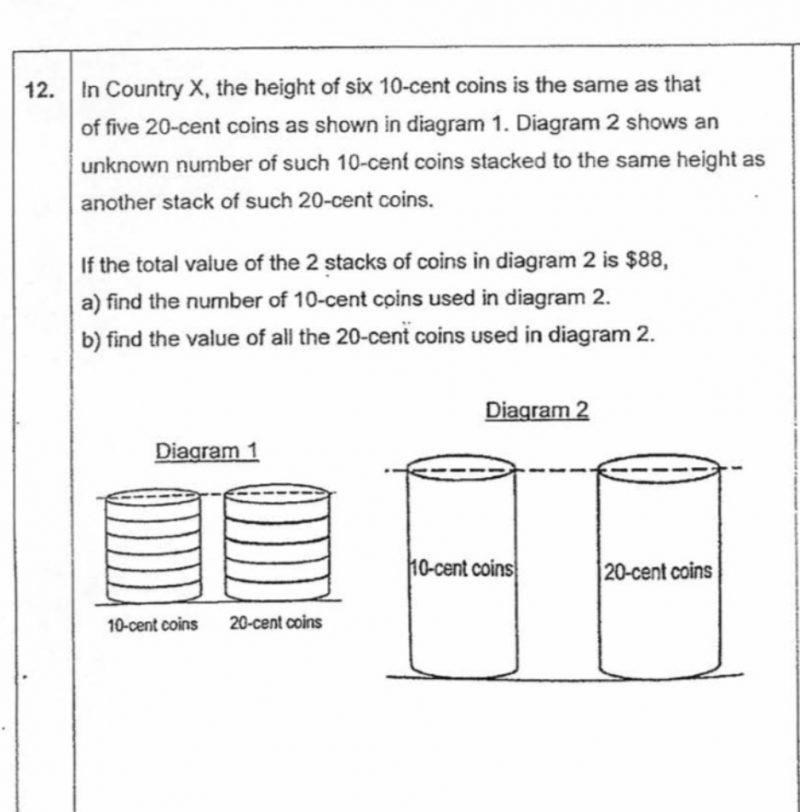# QuestionEvery stack in diagram 1:
10-cents coin -> \$0.60
20-cents coin -> \$1
Total: \$1.60

How many stack in diagram 2:
\$88 / \$1.60 = 55 stacks

(a)
Each stack of 10-cents coin have 6 coins
Therefore, there are 6 coins x 55 stacks = 330 10-cents coin

(b)
Each stack is \$1.
There are 55 stacks.
Therefore, total there are \$1 x 55 stacks = \$55